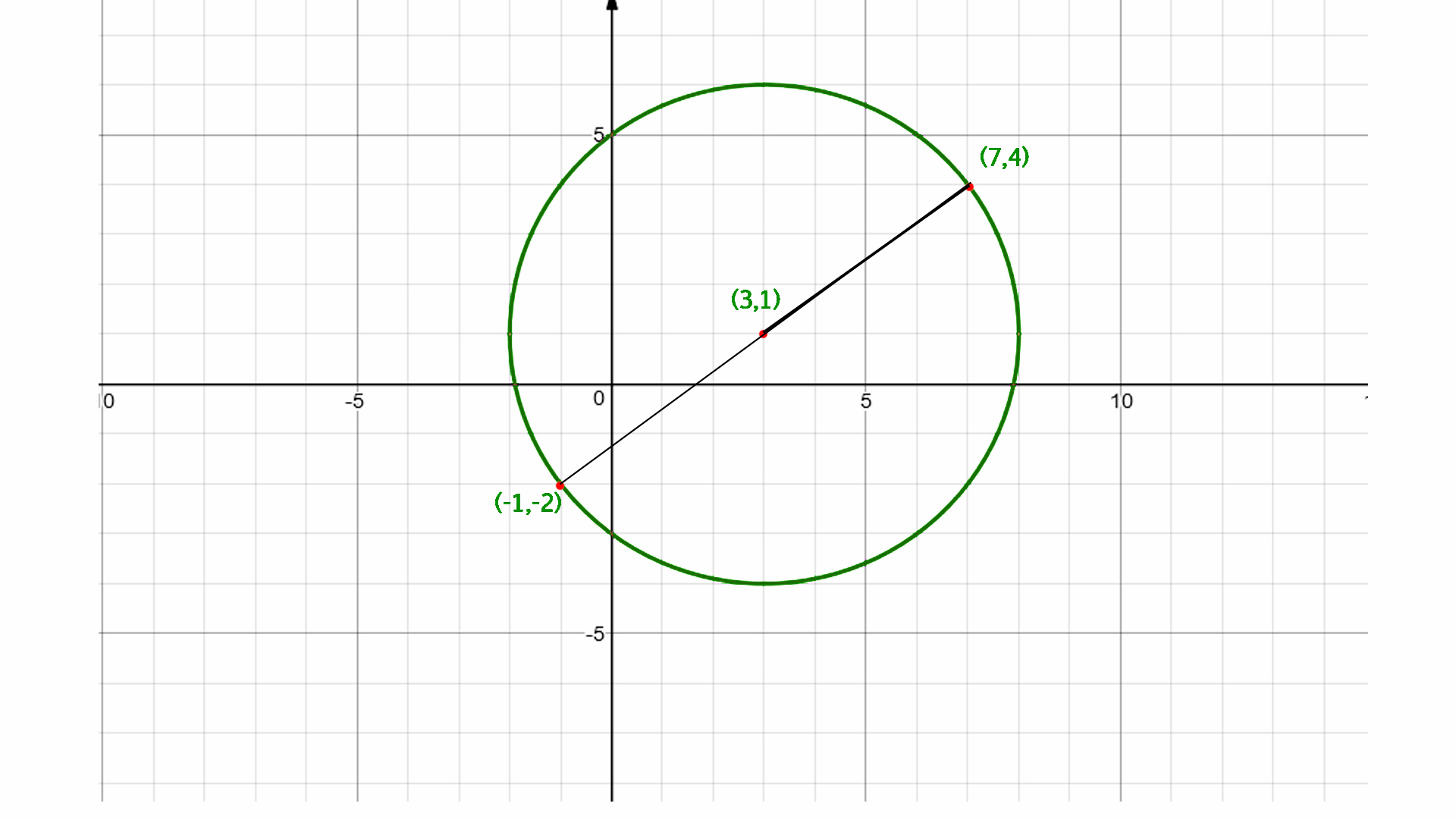Find the center of the circle using endpoints of diameter

• Last Updated : 24 Nov, 2021

Given two endpoint of diameter of a circle (x1, y1) and (x2, y2) find out the centre of a circle.
Examples :

Input  : x1 = -9, y1 = 3, and
x2 = 5, y2 = –7
Output : -2, –2

Input  :  x1 = 5, y1 = 3 and
x2 = –10 y2 = 4
Output : –2.5, 3.5

Midpoint Formula:
The midpoint of two points, (x1, y2) and (x2, y2) is : M = ((x 1 + x 2) / 2, (y 1 + y 2) / 2)
The centre of the circle is the mid point of its diameter so we calculate the mid point of its diameter by using midpoint formula.C++

 // C++ program to find the// center of the circle#include using namespace std;  // function to find the// center of the circlevoid center(int x1, int x2,            int y1, int y2){          cout << (float)(x1 + x2) / 2 <<           ", " << (float)(y1 + y2) / 2;}  // Driven Programint main(){    int x1 = -9, y1 = 3, x2 = 5, y2 = -7;    center(x1, x2, y1, y2);    return 0;}

Java

 // Java program to find the// center of the circleclass GFG {          // function to find the    // center of the circle    static void center(int x1, int x2,                             int y1, int y2)     {                  System.out.print((float)(x1 + x2) / 2             + ", " + (float)(y1 + y2) / 2);    }          // Driver Program to test above function    public static void main(String arg[]) {                  int x1 = -9, y1 = 3, x2 = 5, y2 = -7;        center(x1, x2, y1, y2);    }}  // This code is contributed by Anant Agarwal.

Python3

 # Python3 program to find # the center of the circle  # Function to find the# center of the circledef center(x1, x2, y1, y2) :      print(int((x1 + x2) / 2), end= "")    print(",", int((y1 + y2) / 2) )  # Driver Codex1 = -9; y1 = 3; x2 = 5; y2 = -7center(x1, x2, y1, y2)   # This code is contributed by Smitha Dinesh Semwal

C#

 // C# program to find the// center of the circleusing System;  class GFG {          // function to find the    // center of the circle    static void center(int x1, int x2,                             int y1, int y2)     {                  Console.WriteLine((float)(x1 + x2) / 2                + ", " + (float)(y1 + y2) / 2);    }          // Driver Program to test above function    public static void Main() {                  int x1 = -9, y1 = 3, x2 = 5, y2 = -7;        center(x1, x2, y1, y2);    }}  // This code is contributed by vt_m.



Javascript



Output :

-2, -2

My Personal Notes arrow_drop_up